# MP Board Class 11th Chemistry Solutions Chapter 9 Hydrogen

In this article, we will share MP Board Class 11th Chemistry Solutions Chapter 9 Hydrogen Pdf, These solutions are solved by subject experts from the latest edition books.

## MP Board Class 11th Chemistry Solutions Chapter 9 Hydrogen

### MP Board Class 11 Chemistry Hydrogen Textbook Questions and Answers

Question 1.
Justify the position of hydrogen in the periodic table on the basis of its electronic configuration.
Solution.
Position of hydrogen in the periodic table:
Position of hydrogen could not be assigned to it either in Mendeleer’s periodic table or long form of the periodic table because it resembles both alkali metals as well as halogens.
Resemblance with alkali metals:
(i) Electronic configuration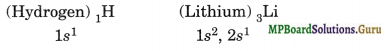(ii) Electropositive character
H → H++ e Li → Li+ + e
(iii) Oxidation state +1
H+Cl Na+Cl Li+Cl K+Br+
(iv) Reaction with electronegative elements or Non-metals
Oxide: H2O, Na2O, Li2O
Halide: HCl, NaCl, KCl
Sulphide: H2S, Na2S
(v) Liberation a the cathode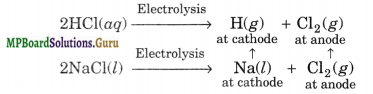(vi) Reducing nature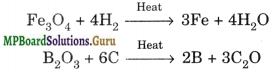Resemblance with halogens
(i) Electronic configuration
(Hydrogen)1H = 1s1 (Fluorine)9F = 1s2, 2s2, 2p5
one e less than He2 One e less than Ne10
(ii) Electronegative nature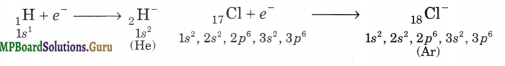(iii) Oxidation state: -1
Na+H Na+Cl
Ca2+H2 Ca++ Cl2
(iv) Liberation at the anode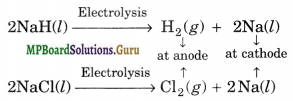(v) Atomicity: Diatomic H2, Cl2, F2, Br2

(vi) Reaction with metals:
2Na + H2 → 2NaH
2Na + Cl2 →2NaCl

(vii) Formation of covalent compounds
with hydrogen: CH4, SiH4, NH3
with halogen: CCl4, SiCl2, NCl3.

Question 2.
Write the names of isotopes of hydrogen. What is the mass ratio of these isotopes?
Solution.
The various isotopes of hydrogen are:
11H(Protium); 21H or D(Deuterium); 13H or T(Tritium)
The mass ratio of 11H: 21H: 13H is 1 : 2 : 3

Question 3.
Why does hydrogen occur in a diatomic form rather than in a monoatomic form under normal conditions?
Solution.
Hydrogen atom has only one electron and thus, to achieve stable inert gas configuration of helium, it shares its single electron with electron of other hydrogen atom to form a stable diatomic molecule. The stability of H2 is further confirmed by the fact, that formation of one mole of gaseous H2 molecules results in the release of 435.8 kJ of energy
H(g) + H(g) → H2(g); ΔH = – 435.8 kJ mol1

Question 4.
How can the production of dihydrogen, obtained from coal gasification, be increased?
Solution.
Coal gasification is the process of producing syngas from coal and steam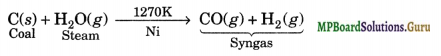The production of hydrogen can be increased by reacting carbon monoxide of syngas with steam in presence of iron chromate as catalyst at 673 K when it is oxidised to CO2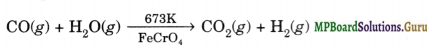The CO2 thus produced is removed by scrubbing with a solution of sodium arsenite.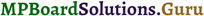Question 5.
Describe the bulk preparation of hydrogen by electrolytic method. What is the role of an electrolyte in this process?
Solution.
(i) By the electrolysis of water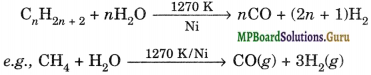(ii) It is obtained as a byproduct in the manufacture of NaOH and Cl2 by the electrolysis of brine solution.
At Cathode:
2H2O(l) + 2e → H2(g) + 2OH(aq)
At anode
2Cl(ag) → Cl2(g) + 2e
2Na+(aq) + 2Cl(aq) + 2H2O → Cl2(g) + H2(g) + 2Na+(aq) + 2OH(aq)
(iii) Reaction of steam on hydrocarbon or coke at high temperatures in the presence of catalyst yields hydrogen.The mixture of CO and H2 is called water gas. It is used for the synthesis of CH3OH and a number of hydrocarbons. It is also called Synthesis gas or Syngas.

Question 6.
Complete the following reactions: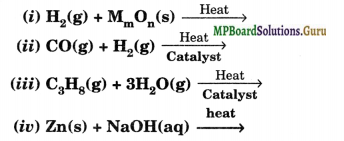Solution.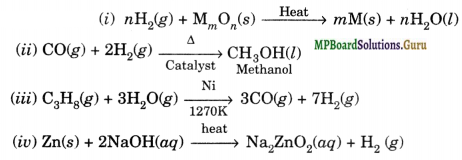Question 7.
Discuss the consequences of high enthalpy of H-H in terms of chemical reactivity.
Solution.
Due to high bond enthalpy of H-H bond, dihydrogen molecule (H2) is quite unreactive at room temperature. However, at high temperature or in presence of catalysts, it combines with many metals and non-metals to form respective hydrides.

Question 8.
What do you understand by (i) electron-deficient, (ii) electron-precise, and
(iii) electron-rich compounds of hydrogen? Provide justification with suitable examples.
Solution.
(i) Hydrides of elements of group-13 such as BH3, AlH3, etc., do not have sufficient number of electrons to form normal covalent bonds and hence are called electron-deficient hydrides. To make up this deficiency, they generally exist in polymeric forms such as B2H6, B4H10, (AlH3)n, etc.

(ii) Hydrides of elements of group-14 like CH4, SiH4, GeH4, etc. have exact number of electrons to form covalent bonds and hence are called electron-precise hydrides. All these hydrides have tetrahedral shapes.

(iii) Hydrides of elements of groups 15, 16 and 17, like NH3, PH3, H2O, H2S, HF, HCl, etc., have more electrons than required to form normal covalent bonds and hence are called electron-rich hydrides. The excess electrons in these hydrides are present as lone pairs of electrons.Question 9.
What characteristics do you expect from electron-deficient hydrides with respect to their structure and chemical reactivity?
Solution.
Electron deficient hydrides do not have sufficient number of electrons to form normal covalent bonds. They generally exist in polymeric forms such as B2H6, B4H10, (AlH3)n etc.
Due to deficiency of electrons, these hydrides act as Lewis acids and thus, form complex entities with Lewis bases such as: NH3, H ions, etc.
B2H6 + 2NH3 → [BH2(NH3)2]+(BH4) OR NH3 → BH3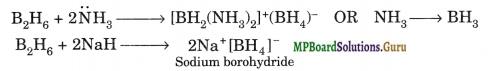Question 10.
Do you expect the carbon hydride of the type (CnH2n+2) to act as Lewis acid or base? Justify your answer.
Solution.
Carbon hydride of the type (CnH2n+2) are electron-precise hydrides. In other words, they have exact numbers of electrons required to form covalent bonds. Therefore, they do not have a tendency to either gain or lose electrons and hence they do not act as Lewis acids or Lewis bases.

Question 11.
What do you understand by the term “non-stoichiometric hydrides”? Do you expect this type of hydrides to be formed by alkali metals? Justify your answer.
Solution.
These are hydrides which have low hydrogen content. In these hydrides the ‘ ratio of the metal to hydrogen atoms is fractional and they are called non-stoichiometric hydrides. Furthermore, even this fractional ratio of atoms is not fixed but varies with the temperature and the pressure conditions. This type of hydrides are formed by d-and /”-block elements. In these hydrides, the hydrogen atoms occupy holes in the metal lattice. Usually some holes always remain unoccupied and hence these metals form non-stoichiometric hydrides.

Alkali metals are highly reducing, they transfer their electrons to the H atom, thereby forming H ions. In other words, alkali metal hydrides are ionic in nature. Since such hydrides are formed by complete transfer of an electron, therefore, the ratio of metal to hydrogen is always fixed. Therefore, alkali metals form only stoichiometric hydrides. They do not form non-stoichiometric hydrides at all.

Question 12.
How do you expect the metallic hydrides to be useful for hydrogen storage? Explain.
Solution.
In some of the transition metal hydrides, hydrogen is absorbed as H-atoms. Due to the inclusion of H-atoms, the metal lattice expands and thus becomes less stable. Therefore, when such metallic hydride is heated, it decomposes to release hydrogen gas and very finely divided metal. The hydrogen evolved in this manner can be used as a fuel. Thus, transition metals or their alloys can act as sponge and can be used to store and transport hydrogen to be used as a fuel.

Question 13.
How does the atomic hydrogen or oxy-hydrogen torch function for cutting and welding purposes? Explain.
Solution.
Atomic hydrogen is produced when molecular hydrogen is passed through an electric arc struck between tungsten electrodes (3773 – 4273K).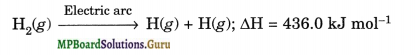The life span of atomic hydrogen is about 0.3 sec and therefore, it immediately gets converted into the molecular hydrogen (H2) liberating a large amount of energy which is used for cutting and welding purposes in form of atomic hydrogen torch.Question 14.
Among NH3, H2O and HF, which would you expect to have highest magnitude of hydrogen bonding and why?
Solution.
Strength of H-bond depends upon the atomic size and electronegativity of the other atom to which H-atom is covalently bonded. Smaller size and higher electronegativity favour H-bonding. Now among N, F, O atoms, atomic size of F is lowest and its electronegativity is highest. Hence, H-F will have highest magnitude of H-bonding.

Question 15.
Saline hydrides are known to react with water violently producing fire. Can, CO2, a well known fire extinguisher, be used in this case? Explain.
Solution.
Saline hydrides (such as NaH, CaH2, etc.), react with water violently to form the corresponding metal hydroxides with the evolution of dihydrogen. The dihydrogen gas so liberated undergoes spontaneous combustion causing fire. This is because of exothermic nature of combustion reactions.
NaH(s) + H2O(l) → NaOH(aq) + H2(g)
CaH2(s) + 2H2O(l) → Ca(OH)2(aq) + 2H2(g)
The fire so produced cannot be extinguished by CO2 because it gets reduced by the hot. metal hydride to formate ions
NaH + CO2→ HCOONa
However, sand (because of its stable nature) is more effective fire extinguisher in such a case.

Question 16.
Arrange the following:
(i) CaH2, BeH2 and TiH2 in order of increasing electrical conductance.
(ii) LiH, NaH and CsH in order of increasing ionic character.
(iii) H-H, D-D and F-F in order of increasing bond dissociation enthalpy.
(iv) NaH, MgH2 and H2O in order of increasing reducing property.
Solution.
(i) BeH2 is a covalent hydride, therefore, it does not conduct electricity at all. CaH2 conducts electricity in the fused state while TiH2 conducts electricity at room temperature. Thus, the order of increasing electrical conductance is: BeH2 < CaH2 < TiH2.
(ii) Electronegativity decreases down the group from Li to Cs, therefore, the ionic char¬acter of their hydrides also increases in the same order, i.e., LiH < NaH < CsH.

(iii) F-F bond dissociation enthalpy is the minimum. This is due to high concentration of electron density around each F atom in the form of 3 lone pairs which cause significant repulsive interactions. The bond enthalpy of D-D bond is slightly higher than that of H-H bond. It is due to marginally smaller size of D. Thus, the bond dissociation enthalpy increases in the order: F-F < H-H < D-D.

(iv) Ionic hydrides are powerful reducing agents. Both MgH2 and H2O are covalent hydrides but the bond dissociation of O-H bonds in H2O is much higher than that of Mg-H bond in MgH2. Therefore, the reducing character increases in the order: H2O < MgH2< NaH.

Question 17.
Compare the structure of H2O and H2O2.
Solution.
Oxygen atom in water assumes sp3 hybridisation. The, two O-H bonds are sp3-s sigma bonds. The HOH bond angle is 104.5°, a little less than idealised angle because of strong lone pair-bond pair repulsions. In H2O2 also each O atom is sp3 hybridised. There is one O-O bond which is sp3-sp3 sigma bonds and two O-H bonds which are sp3-s sigma bonds. The two O-H bonds are, however, present in different planes. The dihedral angle between two planes of O-H bond is 111.5° in gas phase. Thus, the molecule has an open book-like structure.

Question 18.
What do you understand by the term ‘auto-protolysis’ of water? What is its significance?
Solution.
Auto-protolysis refers to self ionization of water. It may be represented as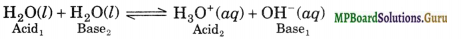Due to auto-protolysis, water acts as amphoteric substance i.e., it reacts with both acids and bases. It acts as a base towards acids stronger than itself and as an acid towards bases stronger than itself. For example,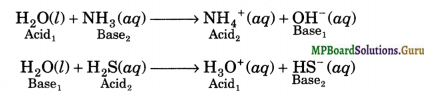Question 19.
Consider the reaction of water with F2 and suggest, in terms of oxidation and reduction which species are oxidised/reduced?
Solution.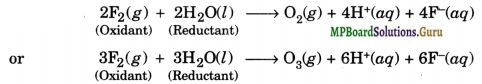In these reactions, water acts as a reducing agent and gets oxidised to either O2 or O3 on the other hand, F2 acts as an oxidising agent, it gets reduced to F ion.

Question 20.
Complete the following chemical reactions:
(i) PbS(s) + H2O2(aq) →
(ii) MnO4(aq) + H2O2(aq) + H+(aq) →
(iii) CaO(s) + H2O(g) →
(iv) AlCl3(g) + H2O(l)→
(iv) Ca3N2(s) + H2O(l) →
Solution.
(i) PbS(s) + 4H2O2(aq) → PbSO4(s) +4H2O(l)
(ii) 2MnO4(aq) +5H2O2(l) +6H+(aq) → 2Mn2+(aq) +8H2O(l) +5O2(g)
(iii) CaO(s) + H2O(g) → Ca(OH)2(aq)
(iv) AlCl3(S) + 3H2O(l) → Al(OH)3(S) + 3HCl(aq)
(v) Ca3N2(s) + 6H2O(l) → 3Ca(OH)2(aq) + 2NH3(aq)Question 21.
Describe the structure of common form of ice.
Solution.
In solid-phase: Ice has a highly ordered three-dimensional hydrogen-bonded structure. In water each hydrogen is covalently bonded to one oxygen atom and linked to another oxygen atom by a hydrogen bond. Such an arrangement leads to a packing with large open spaces. The density of ice is, therefore, less than that of liquid water and hence ice floats over liquid water.

Question 22.
What causes the temporary and permanent hardness of water?
Solution.
Temporary hardness is caused by presence of bicarbonates of calcium and magnesium, i.e., Ca(HCO3)2 and Mg(HCO3)2 in water whereas permanent hardness is caused by presence of soluble chlorides and sulphates of calcium and magnesium, i.e., CaCl2, CaSO4, MgCl2 and MgSO4 in water.

Question 23.
Discuss the principle and method of softening of hard water by synthetic ion- exchange resins.
Solution.
Ion-exchange method: This method is also called zeolite or permutit process. Hydrated sodium aluminium silicate is called zeolite or permutit. When zeolite is added in hard water, exchange reaction takes place, zeolite or permutit represent as Na2Z
Z = Al2Si2O8 . x . H2O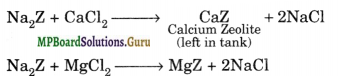Na2Z+ MgCl2 → MgZ + 2NaCl
After sometime the whole of permutit gets exhausted due to the conversion of sodium • Zeolite into calcium and magnesium zeolite. It can, however be regenerated by passing a 10% solution of NaCl through it.
CaZ + 2NaCl → Na2Z + CaCl2
MgZ + 2NaCl → Na2Z + MgCl2
Permutit is an efficient process because it is a very cheap process since during the process only sodium chloride is consumed which is quite cheap and permutit process is used to remove both temporary and permanent hardness completely.

Question 24.
Write chemical reactions to show amphoteric nature of water.
Solution.
Water is amphoteric in character. It means that it can act as proton donor as well as proton acceptor. When it reacts with acids, (stronger than itself), it behaves as a base. When it reacts with bases (stronger than itself), it acts as an acid.Question 25.
Write chemical reactions to justify that hydrogen peroxide can function as an oxidising as well as a reducing agent.
Solution.
H2O2 can act as an oxidising as well as a reducing agent both in acidic and basic media as illustrated below:
(i) Oxidising agent in acidic medium
2Fe2+(aq) + 2H+(aq) + H2O2(aq) → 2Fe3+(aq) + 2H2O(l)
(ii) Oxidising agent in basic medium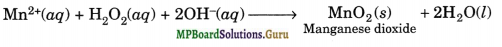(iii) Reducing agent in acidic medium
2MnO4(aq) + 6H+(aq) + 5H2O2(aq) → 2Mn2+
(aq) + 8H2O(l) + 5O2(g)
(iv) Reducing agent in basic medium
2[Fe(CN)6]3-(s) + H2O2(aq) + 2OH(aq) → 2[Fe(CN)6]4- (aq) + 2H2O(l) + O2(g)

Question 26.
What is ‘demineralised water’ and how can it be obtained?
Solution.
Water which is free form all cations and anions is called demineralised water. It is obtained by passing hard water first through a cation exchange resin and then through anion exchange resin. For details refer to section 9.9.

Question 27.
Is demineralised or distilled water useful for drinking purposes? If not, how can it be made useful?
Solution.
Demineralised or distilled water is not useful for drinking purposes because it does not contain even useful minerals. Therefore, to make it useful for drinking pur¬poses, useful minerals in proper amounts should be added to demineralised or distilled water.

Question 28.
Describe the usefulness of water in biosphere and biological systems.
Solution.
Water is essential for all forms of life. It constitutes about 65-70% of the body mass of animals and plants. In comparison to other liquids, water has a high specific heat, thermal conductivity, surface tension, dipole moment and dielectric constant, etc. These properties allow water to play a key role in biosphere.

The high heat of vaporisation and high heat capacity are responsible for moderation of the climate and body temperature of living beings. It is an excellent solvent for transportation of minerals and other nutrients for plant and animal metabolism. Water is also required for photosynthesis in plants which releases O2 into the atmosphere.Question 29.
What properties of water make it useful as a solvent? What type of compounds can it (i) dissolve (ii) hydrolyse?
Solution.
Water has a high dielectric constant (79.39) and high dipole moment (1.84 D). Because of these properties, water dissolves most of the inorganic (ionic) compounds and many covalent compounds. That is why water is called a universal solvent. Ionic compounds dissolve in water due to ion-dipole interactions but, covalent compounds such as alcohol, amines, urea, glucose, sugar, etc., dissolve in water due to H-bonding. Water can hydrolyse many metallic or non-metallic oxides, hydrides, carbides, nitrides, phosphides and other salts. Some of the important hydrolytic reactions are given below.
CaO(s) + H2O(l) → Ca(OH)2(aq)
CaH2(s) + 2H2O(l) → Ca(OH)2(aq) + 2H2(g)
Ca3N2(s) + 6H2O(S)→ 3Ca(OH)2(aq) + 2NH3(g) Ammonia

Question 30.
Knowing the properties of H2O and D2O, do you think that D2O can be used for drinking purposes?
Solution.
Heavy water is injurious to human beings, plants and animals since it slows down the rates of reactions occurring in them. Thus, heavy water does not support life.
In fact, it retards certain cellular process, such as mitosis, cell division, etc. Thus, prolonged use of D2O leads to degeneration of tissues.

Question 31.
What is the difference between hydrolysis and hydration?
Solution.
Hydrolysis refers to the reaction of salt or more precisely ions of the salt to form acidic or basic solution. For example,
Na2CO3 + 2H2O ⇌ 2Na+ + 2OH + H2CO3
NH4Cl + H2O ⇌ H+ + Cl + NH4OH
Hydration, refers to the addition of H2O to ions or molecules to form hydrated ions or hydrated salts. For example,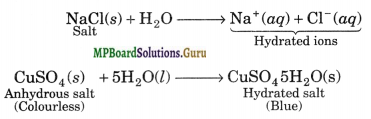Question 32.
How can saline hydrides remove traces of water from organic compounds?
Solution.
Saline hydrides (i.e., NaH, CaH2, etc.) react with water forming their corresponding metal hydroxides with the liberation of H2 gas. Thus, traces of water present in organic solvents can be easily removed by distilling them over saline hydrides when H2 escapes into the atmosphere, metal hydroxide is left in the flask while dry organic solvent distils over.
Alternatively, organic compounds containing traces of water can be dried by placing them in a desiccator containing saline hydrides at the bottom for a few hours or preferably overnight.

Question 33.
What do you expect the nature of hydrides if formed by elements of atomic numbers 15,19,23,44 with dry dihydrogen? Compare their behaviour towards water.
Solution.
(i) Element with Z = 15 is a non-metal (i.e., P) and hence forms covalent hydride (i.e., PH3).
(ii) Element with Z = 19 is a alkali metal (i.e., K) and hence forms saline or ionic hydride (i.e., K+ H).
(iii) Element with atomic number Z = 23 is a transition metal (i.e., V) belonging to group
3. Hence it forms interstitial hydride (i.e., VH1.6).
(iv) Element with Z = 44 is a transition metal (i.e., Ru) belonging to group-8. It does not
form any hydride.
Behaviour towards water. Only ionic hydrides react with water evolving H2 gas.
2KH(s) + 2H2O(S) → 2KOH(ag) + 2H2(g)

Question 34.
Do you expect different products in solution when aluminium (III) chloride and potassium chloride are treated separately with (i) normal water (ii) acidified water, and (iii) alkaline water?
Solution.
KCl is the salt of a strong acid and a strong base. It does not undergo hydrolysis in normal water. It just dissociates to give K+(aq) and Cl(aq) ion giving neutral solution.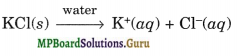AlCl3, on the other hand, is a salt of a weak base Al(OH)3 and a strong acid HCl. Therefore, in normal water, it undergoes hydrolysis, as follows giving acidic solution.
AlCl3(s) + 3H2O(l) → Al(OH)3(s) + H+(ag) + Cl(ag)
In acidic water, both KCl and AlCl3 undergo ionisation.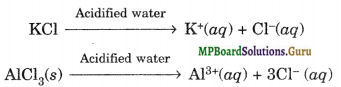In alkaline water, AlCl3 reacts to form soluble tetra hydroxo aluminate complex or meta aluminate ion, i.e.,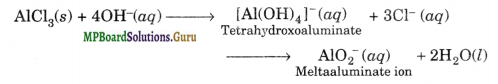KCl does not react and only undergoes ionisation as K+ and C ions.Question 35.
How does H2O2 behave as a bleaching agent?
Solution.
The bleaching action of H2O2 is due to the nascent oxygen which it liberates on decomposition.
2H2O2 →2H2O + 2[O]
The nascent oxygen oxidises the colouring matter to colourless products. It is used for the bleaching of delicate materials like ivory, feather, silk, wool, etc.

Question 36.
What do you understand by the term:
(i) hydrogen economy
(ii) hydrogenation
(iii) syngas
(iv) water gas shift reaction and
(v) fuel cell.
Solution.
(i) Hydrogen economy. Hydrogen economy refers to the proposal to use hydrogen as a fuel in industry, power plants, automobiles and also for domestic needs. The basic principle of hydrogen economy is the transportation and storage of energy in the form of liquid or gaseous dihydrogen.

(ii) Hydrogenation means addition of hydrogen (H2) across double and triple bonds to form saturated compounds. The vegetables oils such as soyabean oil, cottonseed oil, groundnut oil, etc., are called polyunsaturated oils since they contain many C=C bonds in their molecules. When these oils are hydrogenated in presence of finely divided nickel at 473 K they get converted with solid fats due to hydrogenation of C = C bonds.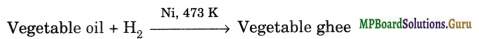This process is called hydrogenation or hardening of oils and is used in the manufacture of vegetable ghee.
(iii) Syngas. The mixture of CO and H2 gases is called synthesis gas or syngas. It can be produced by the reaction of steam on hydrocarbon or coke at high temperature in the presence of nickel as catalyst.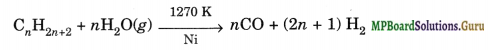These days ‘syngas’ is produced from sewage, saw dust, scrap wood, newspapers, etc. _ The process of producing syngas form coal is called ‘coal gasification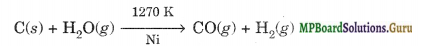(iv) Water-gas shift reaction. This reaction involves the commercial preparation of H2 by selective oxidation of CO of syngas to CO2 with the help of steam in the presence of iron chromate as catalyst.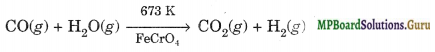(v) Fuel cell is a device which converts the energy produced during the combustion of a fuel directly into electrical energy. Dihydrogen is used in hydrogen-oxygen fuel cells for generating electrical energy. It has many advantages over the conventional fossil fuels. It does not cause any pollution and releases more amount of energy per unit mass of fuel as compared to gasoline and other fuels.

### MP Board Class 11 Chemistry Hydrogen Important Questions and Answers

Question 1.
Can aquatic animals live in distilled water?
No.

Question 2.
Why black suspension of lead sulphide in water decolourises on H2O2?
Due to oxidation of PbS to PbSO4.

Question 3.
How does heavy water differ from ordinary water?
Heavy water is D2O; ordinary water is H2O.

Question 4.
How do calcium carbide and heavy water react?
CaC2 + 2D2O → C2D2 + Ca(OD)2.

Question 5.
What is the mass of 6.02 × 1023 molecules of heavy water (deuterium oxide)?
20 g.

Question 6.
Why cannot H2O2 be stored for prolonged time?
Due to its tendency to decompose
(2H2O2 → 2H2O + O2).

Question 7.
10 ml of H2O2 solution contains 0.91 g of H2O2, what is its strength in volumes?
30 volumes.Question 8.
Perhydrol is the trade name of which chemical species?
Hydrogen peroxide.

Question 9.
Hard water sample is allowed to pass through anion exchange resin, will it produce lather with soap readily?
No because ions causing hardness are still present in it.

Question 10.
Element with atomic number 17 and 20 form compounds with dihydrogen, write the formulae of these compounds and compare their chemical behaviour in water.
HCl, CaH2.

Question 11.
How does heavy water react with Sodium?
2Na + 2D2O → 2NaOD+D2.

Question 12.
Give two important uses of interstitial hydrides.
(i) Storage of H2
(ii) Catalytic agents for hydrogenation.

Question 13.
Give two advantages of using hydrogen as a fuel as compared to gasoline.

1. High enthalpy of combustion and
2. No pollutant as product of combustion.

Question 14.
Give the formulae of two complex metal hydrides which are frequently used as reducing agents in organic chemistry.

1. LiAlH4 and
2. NaBH4.

Question 15.
What is hydrolith? Give its chemical reaction with water.
Hydrolith is CaH2 (Calcium hydride)
CaH2 + 2H2O → Ca(OH)2 + 2H2

Question 16.
How do you account for the solubility of C2H5OH and NaCl in water?
C2H5OH dissolves in water due to its tendency to form H—bonds with water molecules. NaCl dissolves in water due to ion-dipole interactions.

Question 17.
Give the products of electrolysis of H2O and D2O.
H2 and O2; D2 and O2.

Question 18.
Can dihydrogen act as oxidising agent? If so give chemical reactions to support the statement.
Dihydrogen can act as oxidising agent when it forms metal hydrides.Question 19.
Hydrogen can form three types of bonds in its compounds. Describe each type of bonding using suitable examples.
Ionic bond (NaH); Covalent bond (HCl, NH3, H2O) hydrogen bond (NH3, H2O, HF).

Question 20.
Name the class of hydride to which A2O, B2H6, NaH, LaH3 belong.
H2O, B2H6 (covalent), NaH (ionic), LaH3 (interstitial).

Question 21.
Hydrogen peroxide is used to restore the colour of old oil paintings containing lead oxide. Write a balanced equation for the reaction that takes place in this process.
Colour is tarnished due to slow conversion of PbO to PbS by H2S present in atmosphere. H2O2 converts black coloured PbS to PbSO4 which is white.

Question 22.
Complete the following chemical equations.
(i) …………… + H2O → CaCO3 + NH3
(ii) Al4C3 + H2O →………………………
(iii) ……………….. + H2O2 + H+→ CrO5 + ……………………
(i) CaNCN + 3H2O → CaCO3 + 2NH3
(ii) Al4C3 + 12H2O → 4Al(OH)3 + 3CH4
(iii) Cr2O72- + 2H+ + 4H2O2 → 2CrO5 + 5H2O.Question 23.
Complete the following chemical equations.
(i) Mg2+ (aq) + Na2[Na4(PO3)6] →
(ii) …………………… + H2O2 → CaCl2 + H2O + O2
(iii) Na3As + D2O → ………………………..
(i) Mg2+ (aq) + Na2[Na4(PO3)6] → Na2[Mg2(PO3)6](aq) + 4Na+ (aq)
(ii) CaOCl2 + H2O2→ CaCl2 + H2O + O2
(iii) Na3As + 3D2O → 3NaOD + AsD3.

Question 24.
What is the difference between hydrogenation and hydrolysis? Give one example in each case.
Hydrogenation is addition of H2 across multiple bonds.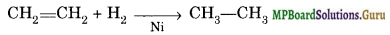Hydrolysis involves breaking of molecules of a substance by water molecules resulting in the formation of new products
2PCl3 + 6H2O → 2H3PO3 + 6HCl

Question 25.
Arrange the following:
(i) CaH2, BeH2 and TiH2 in order of increasing electrical conductance.
(ii) LiH, NaH and CsH in order of increasing ionic character.
(iii) H-H, D-D and F-F in order of increasing bond dissociation enthalpy.
(iv) NaH, MgH2 and H2O in order of increasing reducing property.